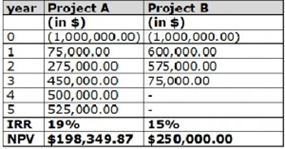Connect faster with

Register

Signup faster with

|   Education without borders.

# Finance: Time Value of Money, Investment Appraisal and Capital Rationing

(1) The Time Value Money is the central concept in finance theory stating that in order to value the investment today, all the future values should be discounted making them all discounted cash flows.

a. State the formula of finding the present value that explains the concept of time value money.

b. What would be the Net Present Value if the expected yearly Cash Inflows are \$300, \$450, \$750 and \$980 assuming the Cost of Capital is \$1,000 and the rate is at 5%?

c. From your answer in (b), explain what you think NPV is?

(2) One of the most important role for a fund manager is to rank the mutually exclusive projects based on the return.  These rankings are important while making investment decisions.  Investment appraisal techniques such as IRR, NPV and PI are used in doing this.

a. Explain briefly in your own words what it means by independent projects and mutually exclusive projects.

b. Explain each investment appraisal techniques and why their rankings are different from one another by answering the questions below:

i.  What is IRR and why is IRR deceiving? (Note: you can answer based on the table given below)ii. What is NPV and why is it used?

iii. What is PI and explain the rule of PI.

(3) Assume that we have a following list of projects with below mentioned cash outflow and their evaluation results based on IRR, NPV and PI along with their respective rankings. The capital ceiling for investment is \$650m.

 Projects Initial   Cash Outflow (\$m) IRR (%) NPV (\$m) PI IRR NPV PI A 350 19 150 1.43 6 2 5 B 300 28 420 2.4 2 1 1 C 250 26 10 1.04 3 6 6 D 150 20 100 1.67 5 5 4 E 100 37 110 2.1 1 4 3 F 100 25 130 2.3 4 3 2

Rank the projects using the three desirable techniques and decide which techniques used will have the highest value and in which selected projects?

(4) Companies may want to implement Capital Rationing in situations where past returns of investment were lower than expected.

a. What are the two ways that companies do rationing?

b. What are the two types of Capital Rationing and explain each type in your words.

c. Explain what it means by Single and Multi-Period Rationing.

5. (a) You are considering a project that costs \$600,000 and has expected cash flows of (in thousand) \$224, \$250.88, and \$280.99 over the next 3 years.  If appropriate discount rate for the project’s cash flows is at 12%, what is the NPV of this project?  (Note: Show your working.)

(b) You are evaluating two mutually exclusive projects with the following net cash flows:

 Year Project A Cash Flow(\$ ,000) Project B Cash Flow(\$ ,000) 0 (100) (100) 1 50 10 2 40 30 3 30 40 4 10 60

If cost of capital is at 15%, which project would you choose and explain your answer.

- Just now

## 0

× Attachments/references, if any, will be shown after refreshing the page.
/homework-help